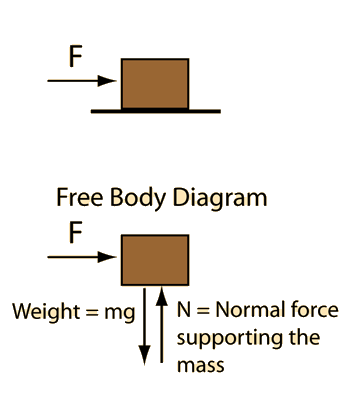# Constant Acceleration MotionApplication of Newton's second law to a single mass.

### Net force = mass x acceleration

For a horizontal applied force F =N.
Net force = N = kg x m/s²

You may edit any of the data. When finished with data entry, click on the quantity you desire to calculate in the formula above. Quantities will not be forced to be consistent until you click on the desired quantity.

In U.S. common units this becomes:
Net force = lb = slug x ft/s²